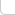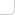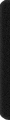# 某岛

… : "…アッカリ～ン . .. . " .. .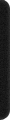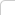May 31, 2014

# SRM 622

## 250. BuildingRoutes

### Brief description:

。问拥挤度 `> K` 的道路有多少。。。

### Analysis:

。。。直接 Floyd + 暴力 O(n4) 枚举统计。。。

## 500. Ethernet

http://hi.baidu.com/thinking610/item/afdd261dd5b152068fbde4d2

## 900. FibonacciXor

### Brief description:

`g(n)` 表示将 `n` 的 Fibnacci 展开示为二进制数时的值。。

———— 例如。。
```9 = F + F = 8 + 1.. g(9) = B(10001) = 17 ```

`\Xor_{i=l}^r g(i)`

。。。`n <= 10^15` ...

```const int FN = 90;
bitset<FN> B[FN], BB;
LL F[FN];

void f(bitset<FN>& b, LL n){
if (n){
int t = upper_bound(F, F+FN, n)-F-1;
if ((n-F[t]+1)&1) b.flip(t);
f(b, n-F[t]); b ^= B[t]; //f(b, F[t]-1);
}
}

class FibonacciXor{
public:
int find(long long l, long long r) {

REP(i, FN) B[i].reset(); BB.reset();

F = 1, F = 2; FOR(i, 2, FN) F[i] = F[i-1] + F[i-2];
FOR(i, 1, FN) f(B[i], F[i]-1);

f(BB, r), f(BB, l-1); int res = 0;
REP(i, FN) if (BB[i]) INC(res, pow(2, i));
return res;
}
};

```

### Analysis:

。。比数位 DP 简单。。直接讨论高位是否是 1。可以递归到两个子问题。。。而其中一个必然是某个 F[i]-1...
。。于是利用 bitset 缓存所有 F[i]-1 位置的结果即可。。